Miscellaneous

Chapter 8 Class 12 Application of Integrals
Serial order wise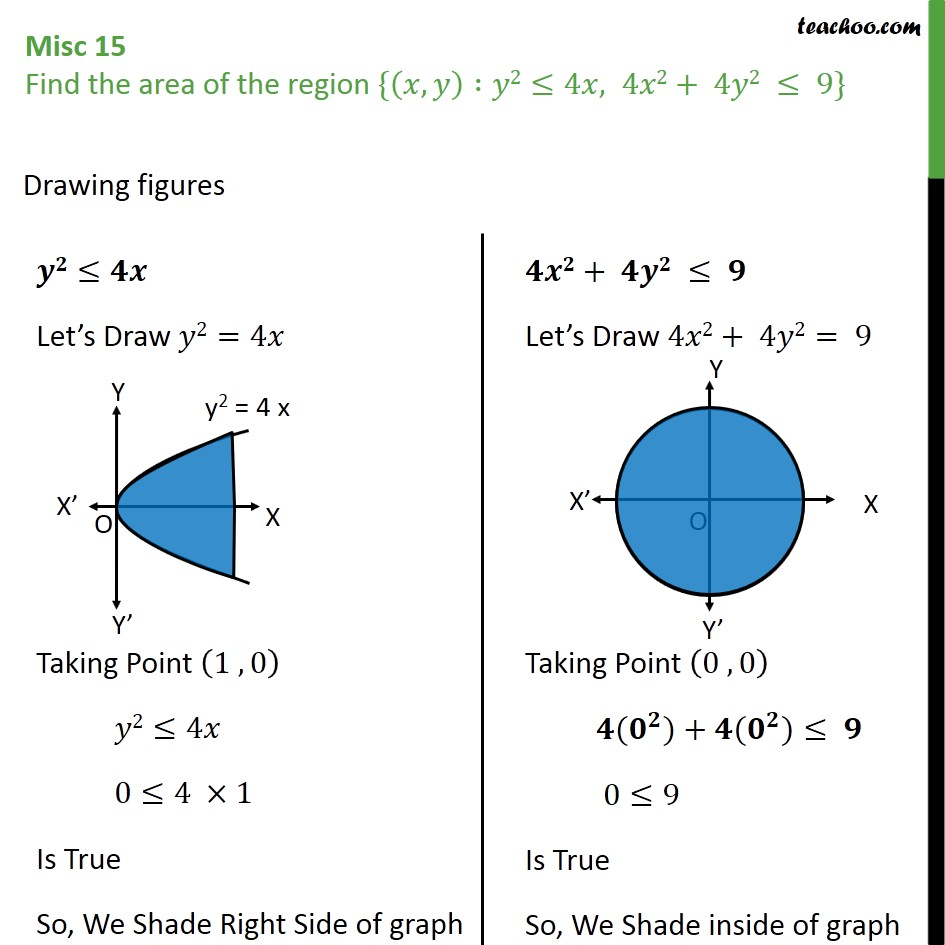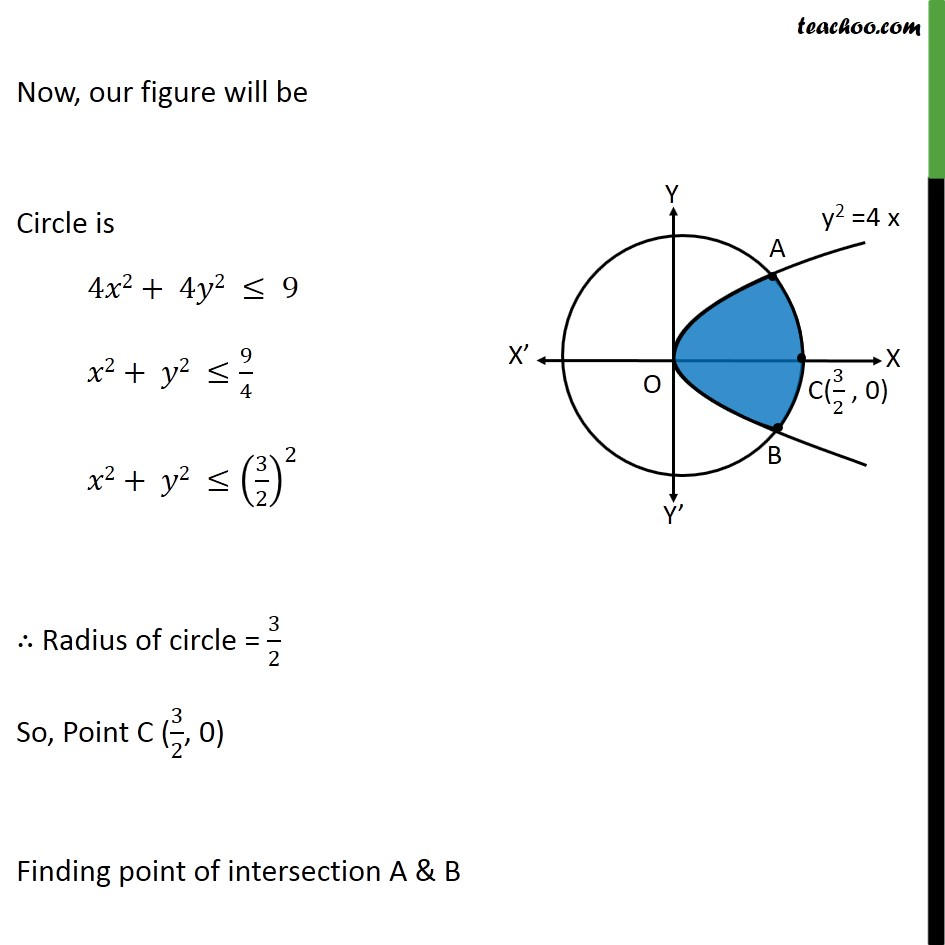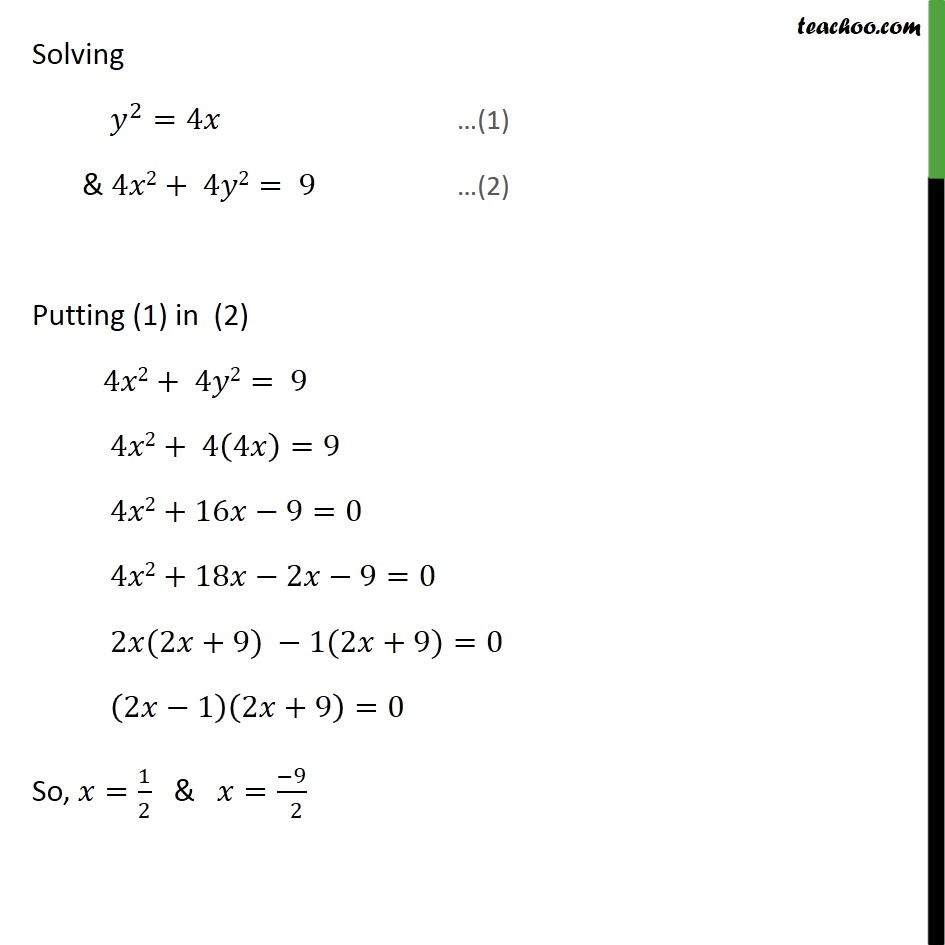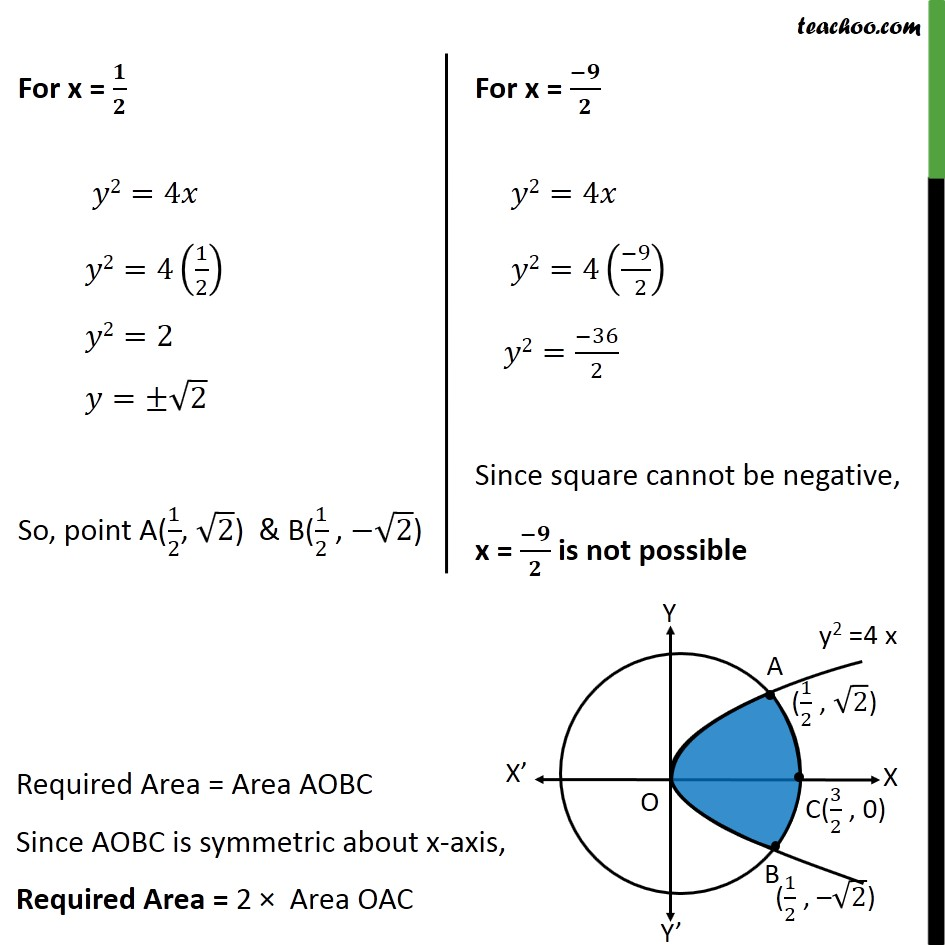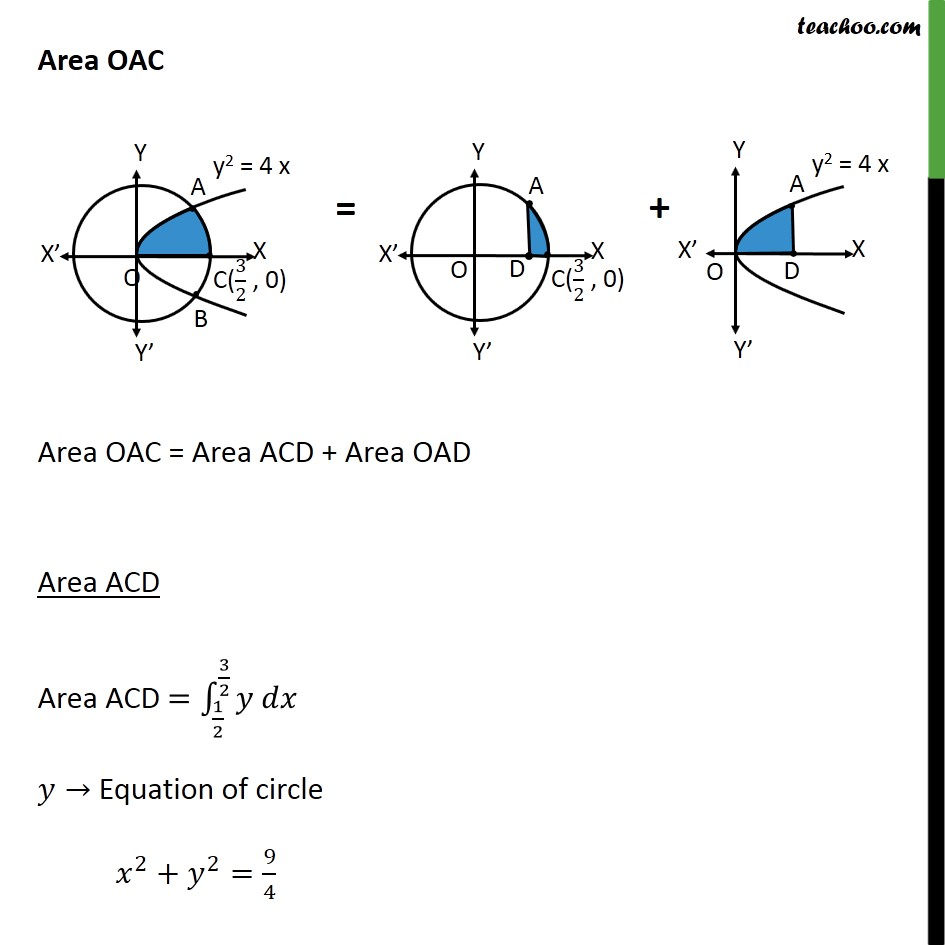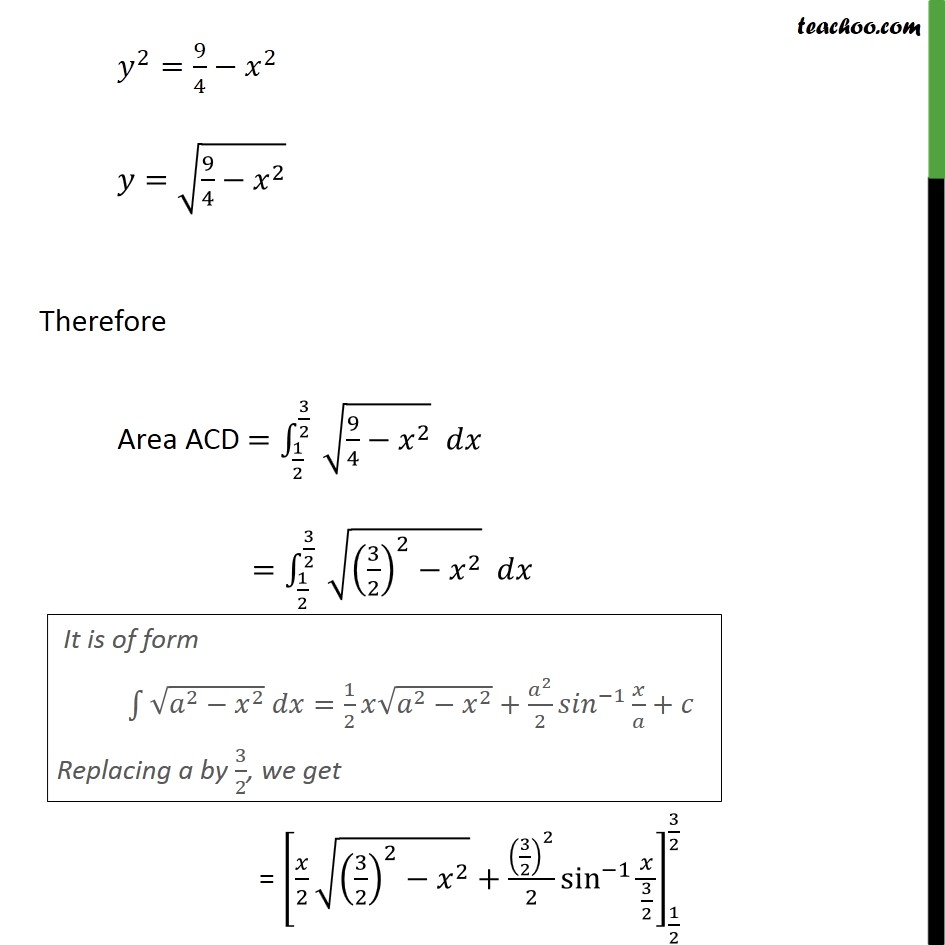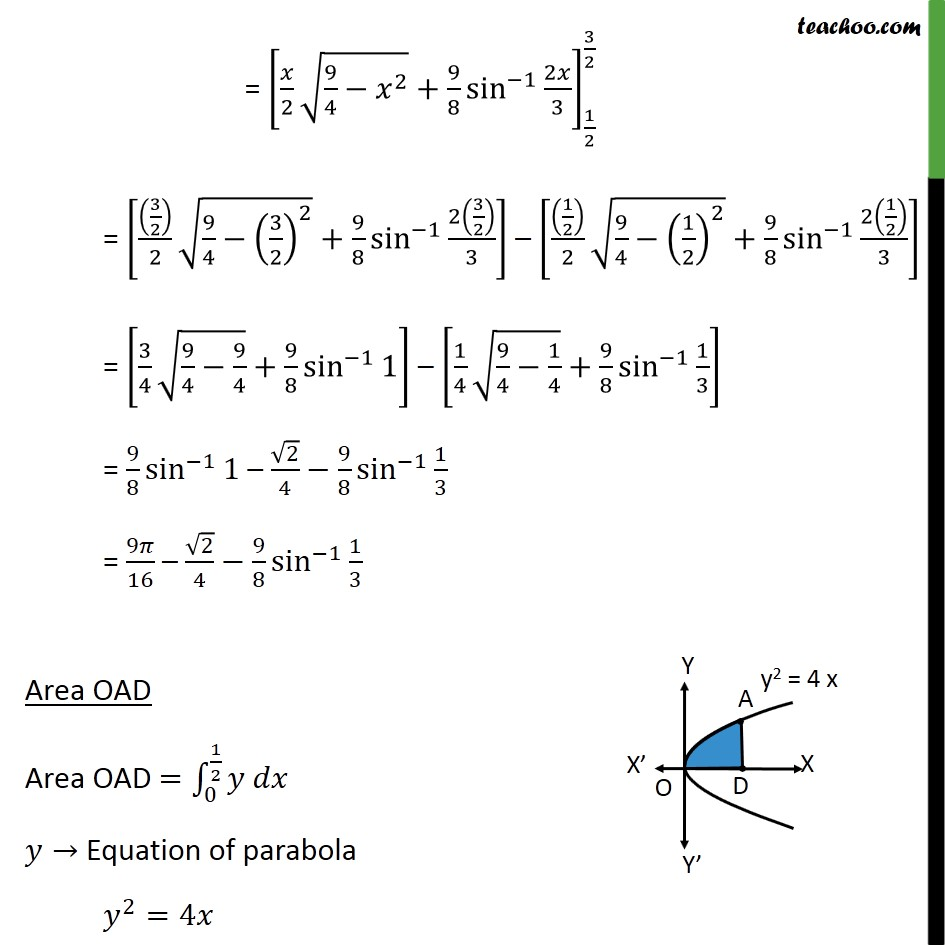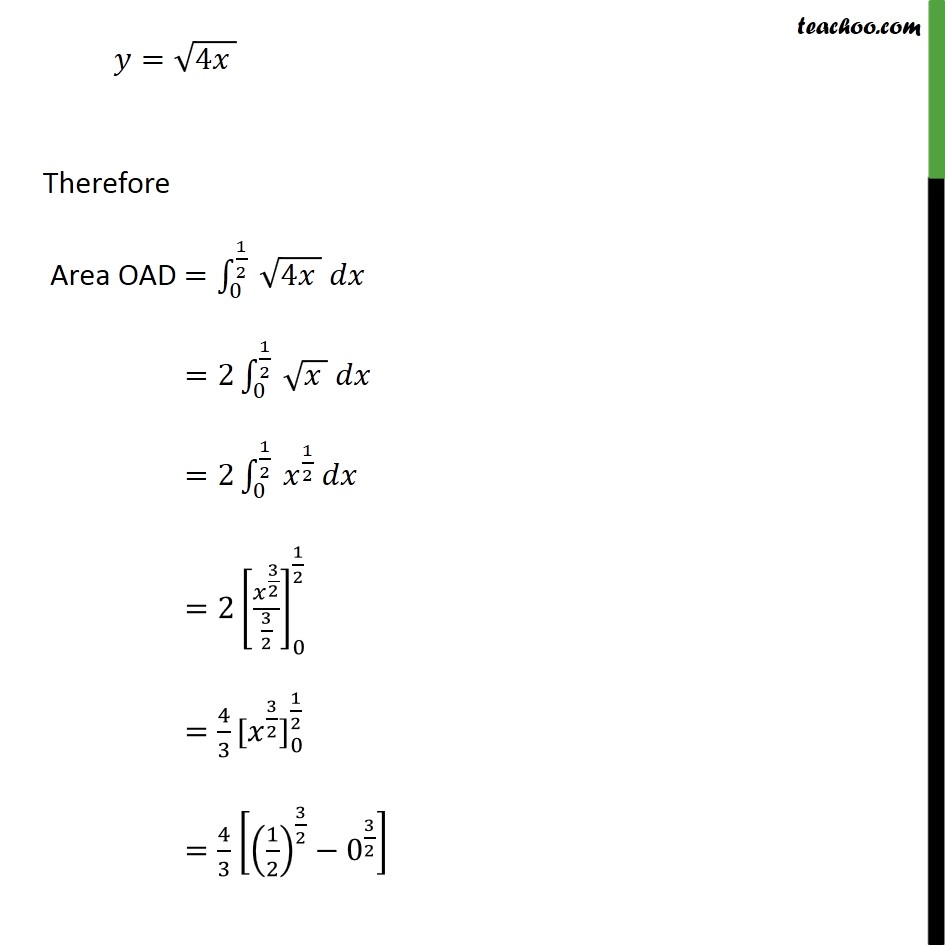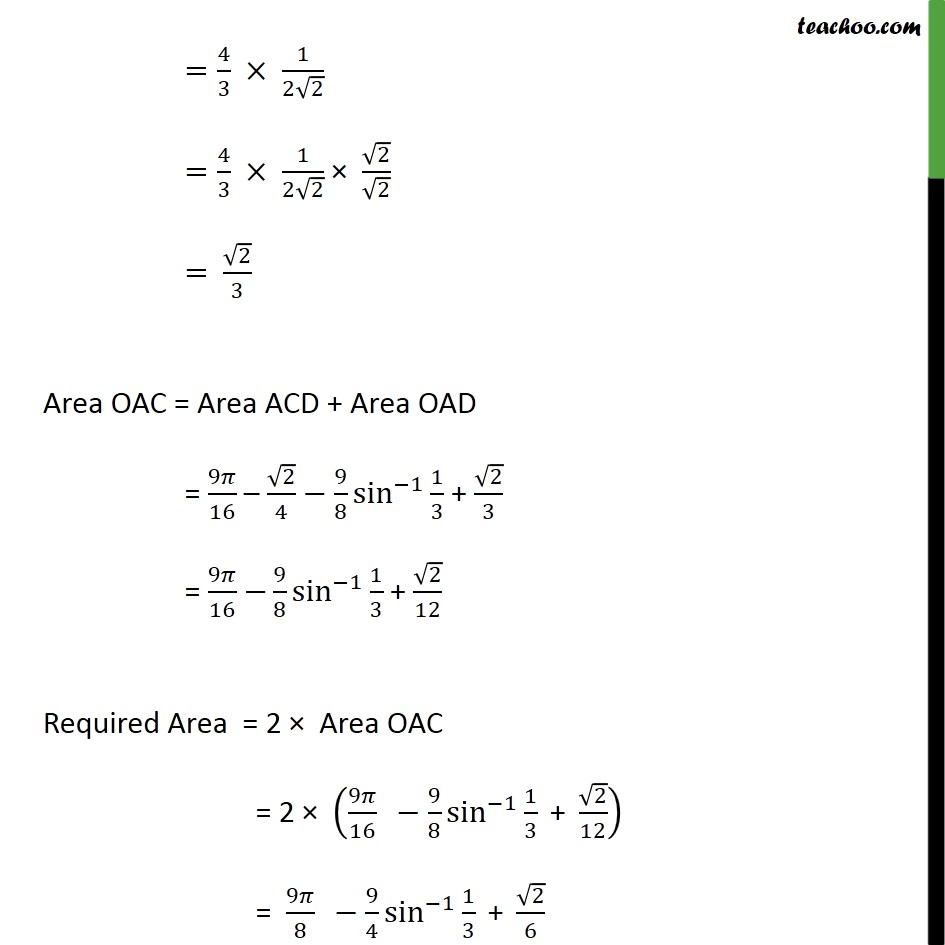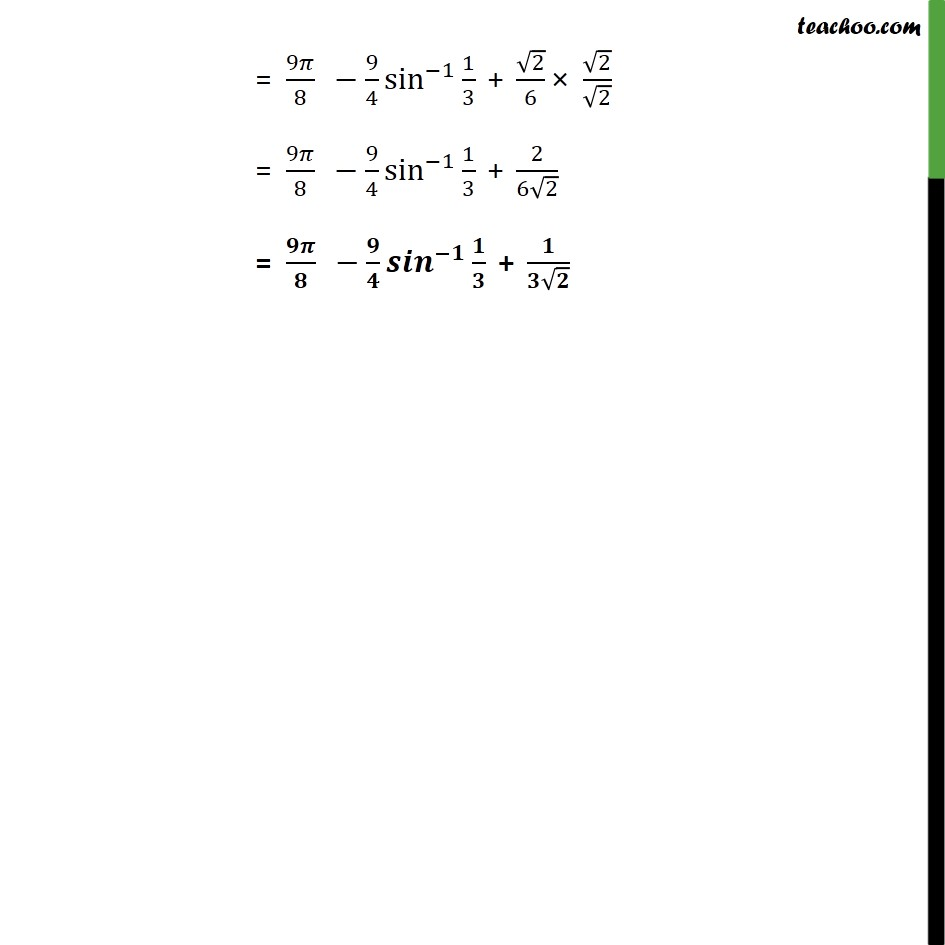Learn in your speed, with individual attention - Teachoo Maths 1-on-1 Class

### Transcript

Question 12 Find the area of the region { , : 2 4 , 4 2+ 4 2 9} Drawing figures Now, our figure will be Circle is 4 2+ 4 2 9 2+ 2 9 4 2+ 2 3 2 2 Radius of circle = 3 2 So, Point C ( 3 2 , 0) Finding point of intersection A & B Solving 2 =4 (1) & 4 2+ 4 2= 9 (2) Putting (1) in (2) 4 2+ 4 2= 9 4 2+ 4 4 =9 4 2+16 9=0 4 2+18 2 9=0 2 (2 +9) 1(2 +9)=0 2 1 2 +9 =0 So, = 1 2 & = 9 2 Area OAC Area OAC = Area ACD + Area OAD Area ACD Area ACD = 1 2 3 2 Equation of circle 2 + 2 = 9 4 2 = 9 4 2 = 9 4 2 Therefore Area ACD = 1 2 3 2 9 4 2 = 1 2 3 2 3 2 2 2 = 2 3 2 2 2 + 3 2 2 2 sin 1 3 2 1 2 3 2 = 2 9 4 2 + 9 8 sin 1 2 3 1 2 3 2 = 3 2 2 9 4 3 2 2 + 9 8 sin 1 2 3 2 3 1 2 2 9 4 1 2 2 + 9 8 sin 1 2 1 2 3 = 3 4 9 4 9 4 + 9 8 sin 1 1 1 4 9 4 1 4 + 9 8 sin 1 1 3 = 9 8 sin 1 1 2 4 9 8 sin 1 1 3 = 9 16 2 4 9 8 sin 1 1 3 Area OAD Area OAD = 0 1 2 Equation of parabola 2 =4 = 4 Therefore Area OAD = 0 1 2 4 =2 0 1 2 =2 0 1 2 1 2 =2 3 2 3 2 0 1 2 = 4 3 [ 3 2 ] 0 1 2 = 4 3 1 2 3 2 0 3 2 = 4 3 1 2 2 = 4 3 1 2 2 2 2 = 2 3 Area OAC = Area ACD + Area OAD = 9 16 2 4 9 8 sin 1 1 3 + 2 3 = 9 16 9 8 sin 1 1 3 + 2 12 Required Area = 2 Area OAC = 2 9 16 9 8 sin 1 1 3 + 2 12 = 9 8 9 4 sin 1 1 3 + 2 6 = 9 8 9 4 sin 1 1 3 + 2 6 2 2 = 9 8 9 4 sin 1 1 3 + 2 6 2 = +